NCERT Solutions for Class 7 Maths Chapter 2 Fractions and Decimals Ex 2.2 are part of NCERT Solutions for Class 7 Maths. Here we have given NCERT Solutions for Class 7 Maths Chapter 2 Fractions and Decimals Ex 2.2.

 Board CBSE Textbook NCERT Class Class 7 Subject Maths Chapter Chapter 2 Chapter Name Fractions and Decimals Exercise Ex 2.2 Number of Questions Solved 8 Category NCERT Solutions

## NCERT Solutions for Class 7 Maths Chapter 2 Fractions and Decimals Ex 2.2

Question 1.
Which of the drawings (a) to (d) show:Solution:
(i) (d)
(ii) (b)
(iii) (a)
(iv) (c)

Question 2.
Some pictures (a) to (c) are given below. Tell which of them show: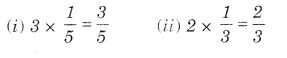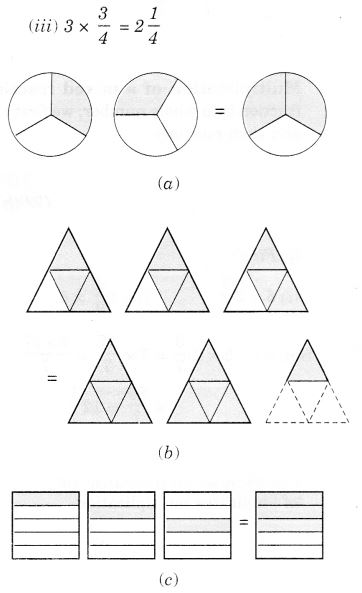Solution: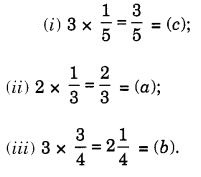Question 3.
Multiply and reduce to lowest form and convert into a mixed fraction: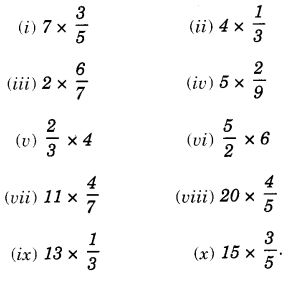Solution: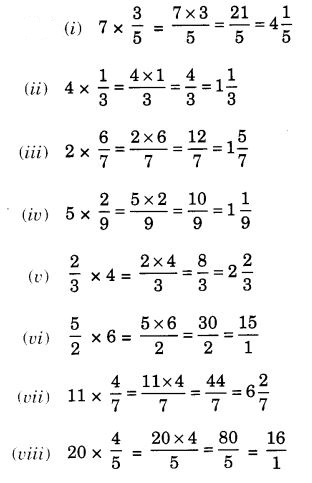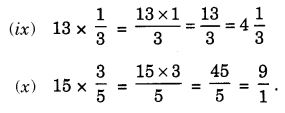Question 4.

1. $$\frac { 1 }{ 2 }$$ of the circles in box (a)
2. $$\frac { 2 }{ 3 }$$ of the triangles in box (b)
3. $$\frac { 3 }{ 5 }$$ of the squares in box (c)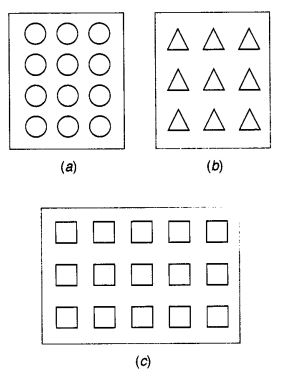Solution: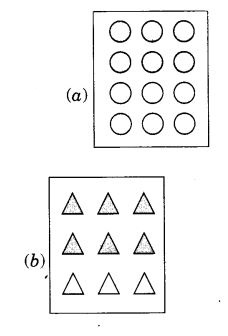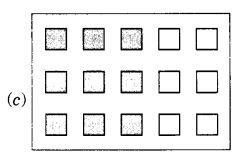Question 5.
Find: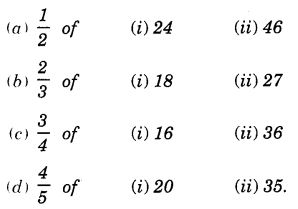Solution: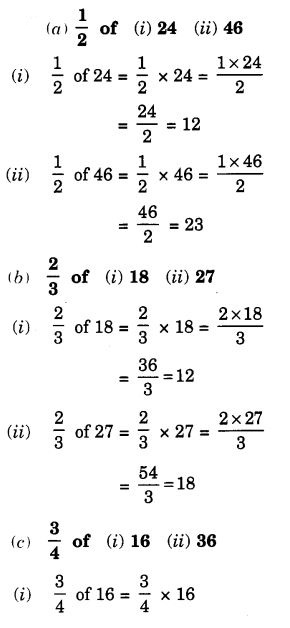Question 6.
Multiply and express as a mixed fraction: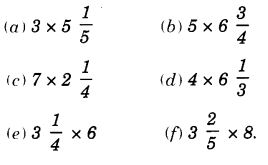Solution: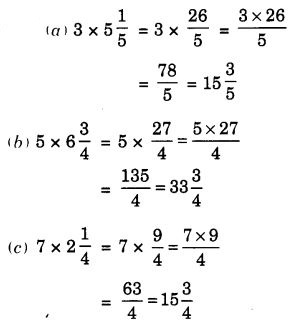Question 7.
FindSolution: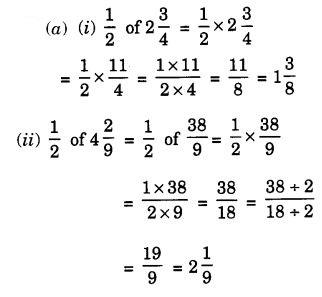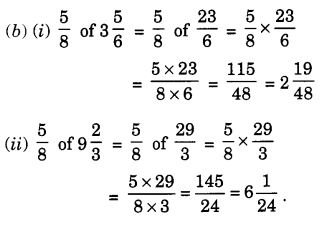Question 8.
Vidya and Pratap went for a picnic. Their mother gave them a water bottle that contained 5 liters of water. Vidya consumed $$\frac { 2 }{ 5 }$$ of the water. Pratap consumed the remaining water.
(i) How much water did Vidya drink?
(ii) What fraction of the total quantity of water did Pratap drink?
Solution:
(i) Quantity of water drank by Vidya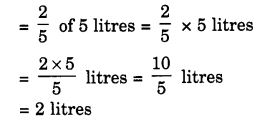(ii) Quantity of water drank by Pratap
= 5 litres – 2 litres = 3 litres
∴ The fraction of the total quantity of water drank by Pratap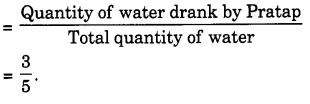We hope the NCERT Solutions for Class 7 Maths Chapter 2 Fractions and Decimals Ex 2.2 help you. If you have any query regarding NCERT Solutions for Class 7 Maths Chapter 2 Fractions and Decimals Ex 2.2, drop a comment below and we will get back to you at the earliest.